Classical Mechanics

# 1D Dynamics: Level 3-5 Challenges

Table shuffleboard is a game (usually found in higher quality bars) in which a heavy puck is glided down a long table toward an end zone. Points are scored by getting the puck to come to rest as close to the edge as possible, the closer the puck, the higher the reward.

If the puck slides off the end of the table, however, the player gets zero points. In the table shown below, three points are awarded for the far zone, one for the near zone, and zero if the puck doesn't cross the first line.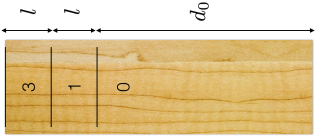Crudely, the difficulty $D$ of making it into each zone is proportional to $1/\Delta s$, the range of allowable speeds between which the puck still stop inside the given zone. For example, if the puck is released with speed $s_3^\textrm{max}$, it will stop just before falling off the end of the table, and if released with speed $s_3^\textrm{min}$, it will stop just inside the three point zone, and $\Delta s_3 = s_3^\textrm{max} - s_3^\textrm{min}$.

Suppose the puck and the table have a coefficient of kinetic friction given by $\mu_k$, and that the puck is pulled down by the acceleration due to gravity, $g$. How much more difficult is it to get the puck to stop in the three point zone than in the one point zone? In other words, find

$\frac{D_3}{D_1} = \frac{\Delta s_1}{\Delta s_3}$

Details

• $d_0=15$ m
• $l=5$ m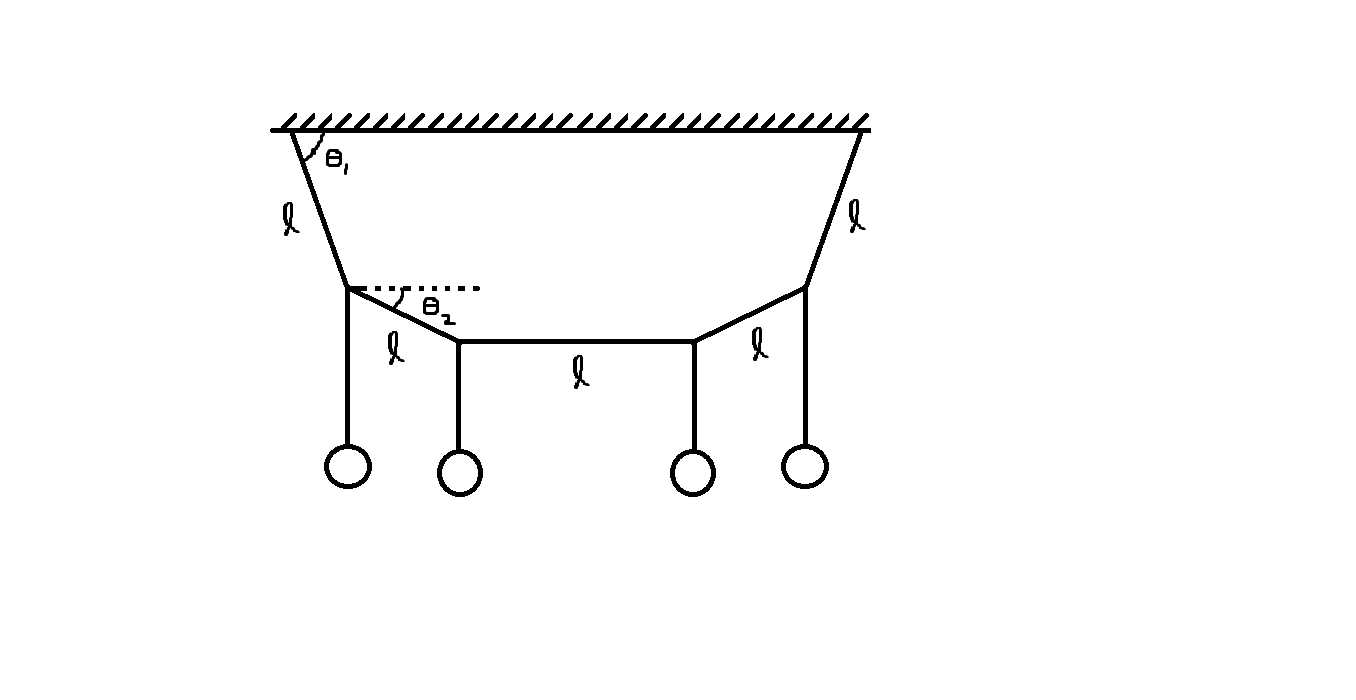Four identical metal balls are hanging from a light string of length $5l$ at equally placed points as shown in figure. The ends of the string are attached to a horizontal fixed support. The middle section of the string is horizontal. Calculate $\theta_2$ in degrees if $\theta_1=\tan^{-1}2$.

###### This problem is a part of My Picks for JEE Mains 1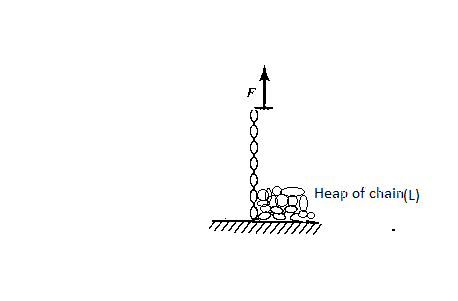A rope of length $l$ and mass density $\lambda$ lies in a heap on a floor. You grab one end of the rope and pull upward with a non-impulsive constant force ${ F }_{ o }$. Find the time period require to completely lift off the rope.

Assume that the rope is greased.

The answer is in the form $\displaystyle{{ T=\cfrac { a }{ g } (\sqrt { \cfrac { { F }_{ o } }{ \lambda } } -\sqrt { \cfrac { { F }_{ o } }{ \lambda } -\cfrac { bgl }{ c } } })}$

Find $a+b+c$

I got this question from Deepanshu.

:D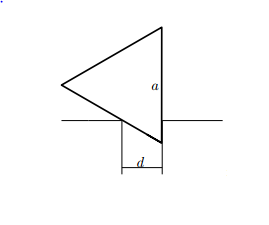A right prism that has an equilateral triangular base with length $a=50cm$ is placed in a horizontal slit between two tables, so that one of the side faces is vertical. How small can the width $d$ of the slit be made in $meters$ before the prism falls out of the slit.

There is no friction between the prism and the tables and the prism is made of a homogeneous material.

The edges of the slit are parallel

This was a past IPHO problem.

When tearing a sheet of paper most people exert a force perpendicular to the sheet, rather than grabbing the sheet by the edges and trying to pull it apart. The physics of this can be understood with a simple model.

Consider a row of three springs along the x-axis with the same spring constant and length, connected end to end. Initially, they are all at their natural length. If any of them stretch by more than one percent they will break. This is our paper.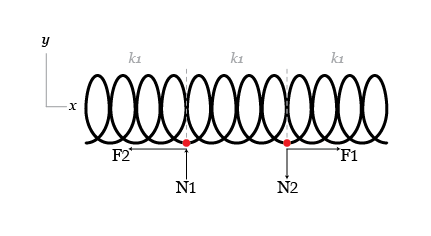Tearing apart is equivalent to putting forces N1 and N2 (each with magnitude N) at the two points where two springs join, in opposite directions and perpendicular to the springs. Pulling apart is equivalent to putting equal and opposite forces F1 and F2 (magnitude F) at the same points but now parallel to the springs. Find the ratio $F/N$ when the middle spring breaks.

Details and assumptions

• Hint 1: Do NOT assume that the ends of the springs move only vertically.
• Hint 2: tear the paper slowly and think about force balances.
×